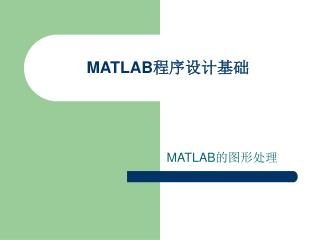DownloadDownload PresentationMATLAB 程序设计基础

# MATLAB 程序设计基础

Download Presentation## MATLAB 程序设计基础

- - - - - - - - - - - - - - - - - - - - - - - - - - - E N D - - - - - - - - - - - - - - - - - - - - - - - - - - -
##### Presentation Transcript

1. MATLAB程序设计基础 MATLAB的图形处理

2. 二维图形 • 基本绘图命令 • plot(Y) Y为实向量，则以Y的索引坐标作为横坐标，Y作为纵坐标来绘制图形。 例： ?y=rand(100,1); ?plot(y)

3. 如果Y为复数向量，则以向量的实部作为横坐标，虚部作为纵坐标，来绘制图形。如果Y为复数向量，则以向量的实部作为横坐标，虚部作为纵坐标，来绘制图形。 例： ?x=rand(100,1); ?z=x+y.*i; ?plot(z)

4. plot(X,Y) 第一个变量作为横坐标，第二个变量作为纵坐标。 例： ?x=0:0.01*pi:pi; ?y=sin(x).*cos(x); ?plot(x,y) 注：两个向量必须维数相同且同为行向量或列向量。

5. 当X和Y为同阶矩阵时，按照矩阵的行或列操作，可以在同一幅图中绘出所有曲线。当X和Y为同阶矩阵时，按照矩阵的行或列操作，可以在同一幅图中绘出所有曲线。 例： ?x=0:0.01*pi:pi; ?y=[sin(x'),cos(x')]; ?plot([x',x'],y);

6. plot(X,Y,s) s参数： - 实线 . 点 * 星号 ^ 上三角 ： 点线 o 圆 s 方形 < 左三角 -. 点划线 x x符号 d 菱形 > 右三角 -- 虚线 + +号 v 下三角 p 正五边形 y 黄色 m 紫红色 c 蓝绿色 r 红色 g 绿色 b 蓝色 w 白色 k 黑色 上述参数的不同组合可以为图形设置不同的线形、颜色和标识，调用时以单引号来引用，各选项直接相连，不需要分隔符。

7. 例： ?x=0:0.1*pi:pi; ?y=sin(x); ?z=cos(x); ?plot(x,y,'--k',x,z,'-.rd')

8. 特殊二维图形函数 • 特殊坐标系的二维函数 • 对数坐标 semilogx、semilogy、loglog格式与plot类似。 例： ?x=1:0.1*pi:2*pi; ?y=sin(x); ?semilogx(x,y,'-*')

9. 极坐标系 polar(theta,rho) polar(theta,rho,s) 其中，theta为角向量，rho为幅向量，s为图形属性选项。 例： ?x=1:0.01*pi:4*pi; ?y=sin(x/2)+x; ?polar(x,y,'-')

10. 双y轴坐标系 plotyy(X1,Y1,X2,Y2) plotyy(X1,Y1,X2,Y2,fun) plotyy(X1,Y1,X2,Y2,fun1,fun2) 其中，fun、fun1、fun2可以为plot、semilogx、semilogy、loglog等。 例： ?x=1:0.1*pi:2*pi; ?y=sin(x); ?z=exp(x); ?plotyy(x,y,x,z,'plot','semilogy')

11. 二维特殊函数图 area 填充绘图 fplot 函数图绘制 bar 条形图 hist 直方图 barh 水平条形图 pareto Pareto图 comet 彗星图 pie 饼状图 errorbar 误差带图 plotmatrix 分散矩阵图 ezplot 简单绘制函数图 ribbon 三位图的二维条状显示 ezpolar 简单绘制极坐标图 scatter 散射图 feather 矢量图 stem 离散序列饼状图 fill 多边形填充 stairs 阶梯图

12. 例：绘制条形图 ?x=1:10; ?y=rand(10,1); ?bar(x,y); 例：绘制矢量图 ?x=0:0.1*pi:2*pi; ?y=x.*sin(x); ?feather(x,y)

13. 例：函数图形绘制 ?lim=[0,2*pi,-1,1]; ?fplot('[sin(x),cos(x)]',lim)

14. 例：绘制饼图 ?x=[2 4 6 8]; ?pie(x)

15. 绘制等高线图 contour(Z,N/V) contour(X,Y,Z,N/V) N为所绘等高线的条数，V为输入向量，等高线的值为对应向量的元素值。 例： ?a=rosser; ?v=[-1000,-500,-100,0,100,500,1000]; ?contour(a,v) ?contourf(a,v)

16. 三维图形 • 基本绘图命令 • plot3函数 plot3(x,y,z) x,y,z为相同维数的向量，绘出这些向量表示的点的曲线。 plot3(X,Y,Z) X,Y,Z为相同阶数的矩阵，绘出矩阵列向量的曲线。 plot3(X,Y,Z,s) s为定义线型的字符串。 plot3(x1,y1,z1,s1,x2,y2,s2,…)

17. 例：绘三维螺旋线 ?x=0:pi/50:10*pi; ?y=sin(x); ?z=cos(x); ?plot3(x,y,z);

18. 网图函数 • [X,Y]=meshgrid(x,y) x,y为给定向量，用来定义网格的划分区域和划分方法。矩阵X和Y为网格划分后的数据矩阵。 例： ?[x,y]=meshgrid(-3:0.1:3,-2:0.1:2); ?z=(x.^2-2*x).*exp(-x.^2-y.^2-x.*y); ?plot3(x,y,z)

19. mesh(X,Y,Z)绘制彩色网格面图形 • mesh(x,y,Z) x,y为两个向量，要求 [length(y),length(x)]=size(Z) • mesh(Z) [m,n]=size(Z), 则使用x=1:n及y=1:m 例： ?x=-8:0.5:8;y=x'; ?a=ones(size(y))*x; ?b=y*ones(size(x)); ?c=sqrt(a.^2+b.^2)+eps; ?z=sin(c)./c; ?mesh(z)

20. meshc与mesh调用方式相同，且能绘制相应的等高线。 meshc与mesh调用方式相同，且能绘制相应的等高线。 例： ?[x,y]=meshgrid([-4:0.5:4]); ?z=sqrt(x.^2+y.^2); ?meshc(z)

21. meshz与mesh调用方式相同，增加了边界面屏蔽。 例： ?[x,y]=meshgrid([-4:0.5:4]); ?z=sqrt(x.^2+y.^2); ?meshz(x,y,z)

22. 着色函数 surf(X,Y,Z) 绘制着色的三维表面图，参数设置与mesh相同。 例： ?[x,y]=meshgrid([-4:0.5:4]); ?z=sqrt(x.^2+y.^2); ?surf(x,y,z)

23. 三维等高线 例： ?waterfall(z) ?[x,y]=meshgrid([-4:0.5:4]); ?contour3(peaks(x,y),25);

24. 特殊三维图形函数 bar3 三维条形图 surfc 着色与等高线结合 comet3 三维彗星轨迹图 trisurf 三角形表面图 ezgraph3 函数控制绘制三位图 trimesh 三角形网格图 pie3 三维饼状图 waterfall 瀑布图 scatter3 三维散射图 cylinder 柱面图 stem3 三维离散数据图 sphere 球面图

25. 绘三维饼图 例： ?x=[2 4 6 8]; ?pie3(x,[0,0,1,0])

26. 柱面图: [X,Y,Z]=cylinder(R,N) R为母线向量，N为旋转圆周上分割线的条数。 例：?x=0:pi/20:pi*3; ?r=5+cos(x); ?[a,b,c]=cylinder(r,30); ?mesh(a,b,c)

27. 图形处理常用技术 • 图形控制 • 坐标轴控制函数 axis(V) V为坐标轴范围数组： 二维图形：V=[XMIN,XMAX,YMIN,YMAX] 三维图形：V=[XMIN,XMAX,YMIN,YMAX,ZMIN,ZMAX]

28. axis ‘控制字符串‘ auto 自动模式 axis 当前坐标设置固定 equal 控制各坐标轴的分度使其相等 square 使绘图区为正方形 normal 解除对坐标轴的任何限制 …

29. 坐标轴缩放函数 zoom ’控制字符串‘ 实现对二维图的缩放 on 允许对图形缩放 off 禁止对图形缩放 (factor) 以factor为缩放因子进行坐标轴缩放 out 恢复所进行的一切缩放 xon 只允许对x轴进行缩放 yon 只允许对y轴进行缩放

30. 平面坐标网格函数 grid on/off显示和取消网格 • 坐标轴封闭函数 box on/off四周显示坐标轴和常规坐标轴

31. 图形的标注 • 坐标轴标注 title xlabel ylabel(‘标注’,’属性1’,属性值1,…) 例： • ?x=0:0.1*pi:2*pi; • ?y=sin(x); • ?plot(x,y) • ?xlabel('x(0~2\pi)','FontWeight','bold'); • ?ylabel('y=sin(x)','FontWeight','bold'); • ?title('正弦函数','Fontsize',12,'fontweight','bold','fontname','黑体')

32. 特殊符号的输入 转义符号\ \alpha \Gamma \beta \Delta \gamma \Theta \delta \Lambda \epsilon \Xi \zeta \Pi \eta \omega \theta …

33. 标注的显示控制 \bf 黑体 \it 斜体 \rm 标准形式 \fontname{fontname} 字体名 \fontsize(fontsize) 字体大小 特殊符号如”\”、”{“、”^“等，用”\”来引导。

34. 上标和下标 调用符为”_”和”^”,相应的内容置于{ }内。 例： ?x=-10:0.1:10; ?y=exp(-x.^2/2); ?title('\bf y=e^{-x^{2}/2}')

35. 文本标注 text(x,y,’标注文本及控制字符串’) (x,y)为给定标注文本在途中添加的位置。 例： ?x=1:0.1*pi:2*pi; ?y=sin(x); ?plot(x,y); ?text(3*pi/4,sin(3*pi/4),'\leftarrow sin(3\pi/4)=-7.707')

36. 复杂文本标注 例： ?x=1:0.1*pi:2*pi; ?y=sin(x); ?plot(x,y); ?text(3*pi/4,sin(3*pi/4),['\leftarrow sin(3\pi/4)=',num2str(sin(3*pi/4))],'fontsize',12) ?text(5*pi/4,sin(5*pi/4),['sin(5\pi/4)=',num2str(sin(5*pi/4)),'\rightarrow'],... 'horizontalalignment','right','fontsize',12)

37. 交互式文本标注 gtext(s) 执行时，图形中出现“十”字型交叉线供用户选择标注位置。S为标注内容的字符串，标注内容为多行时，s为一单元型字符数组。

38. 图例标注 legend(‘标注1’，‘标注2’，…) 标注1、标注2等分别对应绘图过程中绘制的先后顺序的曲线。 例： ?x=1:0.1*pi:2*pi; ?y=sin(x); ?z=cos(x); ?plot(x,y,x,z) ?legend('sin(x)','cos(x)')

39. legend(‘标注’,…,’定位代号’) 定位代号： 0 自动定位 1 右上角 2 左上角 3 左下角 4 右下角 －1 右外侧

40. 图形的保持和子图 • 图形的保持 hold on启动图形保持功能，此后所有的图形都添加到当前的图形窗口中，系统会自动调整坐标。 hold off关闭图形保持功能。 例： ?x=0:0.1*pi:2*pi; ?y=sin(x); ?z=cos(x); ?plot(x,y,'-*') ?hold on ?plot(x,z,'-o') ?plot(x,y+z,'-h') ?legend('sin(x)','cos(x)','sin(x)+cos(x)',0) ?hold off

41. 子图 subplot(m,n,p) 将一个图形窗口分隔成m×n格区域，参数p指定子绘图区域。 例： ?x=0:0.1*pi:2*pi; ?subplot(2,2,1); ?plot(x,sin(x),'-*') ?title('sin(x)') ?subplot(2,2,2); ?plot(x,cos(x),'-o') ?title('cos(x)') ?subplot(2,2,3); ?plot(x,sin(x).*cos(x),'-x') ?title('sin(x)*cos(x)') ?subplot(2,2,4); ?plot(x,sin(x)+cos(x),'-h') ?title('sin(x)+cos(x)')

42. 图形处理的高级技术 • 颜色 采用RGB色系，由矩阵[R,G,B]来表示颜色的映像。

43. 常用的颜色映像（默认状态为64×3颜色矩阵）

44. 着色过程 • 生成颜色映像 colormap(c) c为颜色映像矩阵，3列，行数不限 • 图形应用函数 如pcolor、colorbar等 • 着色方式 shading ‘c’ c为控制字符串，分别为faceted、interp和flat。其中faceted以平面作为着色单位，为默认形式；interp以插值形式为图形像点着色；flat以平滑形式着色。

45. pcolor(c) 以当前的颜色映像为矩阵c进行着色。 例： ?colormap(hot(80)) ?pcolor(rand(6,8)); ?shading interp

46. rgbplot(c) 将矩阵的三列值分别以红、绿、蓝绘出。

47. colorbar(‘vert’) %垂直显示颜色标尺 • colorbar(‘horiz’) %水平显示颜色标尺 例： ?[x,y,z]=peaks; ?mesh(x,y,z); ?colormap(hot(80)); ?colorbar

48. brighten(beta) beta大于零时，增加当前的色彩强度；相反，当beta小于零时，削弱当前的颜色强度。

49. caxis([cmin,cmax]) • [cmin,cmax]=caxis 函数自动控制图形中色彩和数值的对应关系。 • colordef color none ％将图形背景与窗口背景设为相同 color black ％将图形背景设为黑色 color white ％将图形背景设为白色

50. 视觉与光照 • 视觉控制 • view(az,el) az为方位角，即xy平面旋转的角度，顺时针方向旋转的角度为正；el为仰角，即从xy平面向z轴旋转的角度，朝向z轴的旋转（仰视）为正角度；单位均为度，而非弧度。 • view(2)二维图形中视角的默认值(0,90) • view(3)三维图形中视角的默认值(-37.5,30) • [az,el]=view返回当前图形的视角。